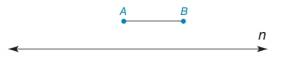Chapter 1.3, Problem 31E### Elementary Geometry for College St...

6th Edition
Daniel C. Alexander + 1 other
ISBN: 9781285195698

#### Solutions

Chapter
Section### Elementary Geometry for College St...

6th Edition
Daniel C. Alexander + 1 other
ISBN: 9781285195698
Textbook Problem
1 views

# In Exercises 29 to 32, use only a compass and a straightedge to complete each construction. Given: A B ¯ as shown in the figure Construct: P Q ¯ on line n so that P Q = 3 ( A B )Exercises 31, 32

To determine

To find:

The complete construction of PQ¯ on line n so that PQ=3(AB).

Explanation

Given:

The given figure is,

Approach:

The steps are given below for the complete construction.

a) Draw a straight line and label a point P.

b) Use compass and open it to the width of AB¯. Use P as center, mark the measured distance and label the point as 1.

c) Use compass and open it to the width of AB¯. Use 1 as center, mark the measured distance and label the point as 2.

d) Use compass and open it to the width of AB¯

### Still sussing out bartleby?

Check out a sample textbook solution.

See a sample solution

#### The Solution to Your Study Problems

Bartleby provides explanations to thousands of textbook problems written by our experts, many with advanced degrees!

Get Started

#### Find the horizontal and vertical asymptotes of the graph of

Mathematical Applications for the Management, Life, and Social Sciences

#### The unit vector in the direction of is:

Study Guide for Stewart's Multivariable Calculus, 8th

#### Which graph represents y=x+1x

Study Guide for Stewart's Single Variable Calculus: Early Transcendentals, 8th

#### In Exercises 110, find the graphical solution to each inequality. 4x80

Finite Mathematics for the Managerial, Life, and Social Sciences

#### Evaluate each sum. k=341k

College Algebra (MindTap Course List)

#### What is a majority?

Mathematics: A Practical Odyssey